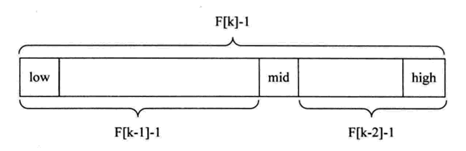七大查找算法, 面试考试皆可用﻿

1、顺序查找

2、二分查找

3、插值查找

4、斐波那契查找

5、树表查找

6、分块查找

7、哈希查找

﻿

1、顺序查找

﻿

﻿

﻿

/**顺序查找平均时间复杂度 O（n）
* @param key 要查找的值
* @param array 数组（从这个数组中查找）
* @return 查找结果（数组的下标位置）
*/
public static int orderSearch(int key,int[] array){
if(array == null || array.length < 1)
return -1;
for(int i = 0; i < array.length; i++){
if(array[i] == key){
return i;
}
}
return -1;
}

2、二分查找

﻿

﻿

//二分查找，递归版本
public static int binarySearch(int array[], int value, int low, int high)
{
if(low>high){
return -1;
}
int mid = low+(high-low)/2;
if(array[mid] == value)
return mid;
if(array[mid] > value)
return binarySearch(array, value, low, mid-1);
if(array[mid] < value)
return binarySearch(array, value, mid+1, high);
}
//二分查找，非递归版本
public static int binarySearch2(int[] array, int key) {
int low = 0;
int high = array.length - 1;
while (low <= high) {
//防止数组越界，且位运算比除法运算快
int mid = low + ((high - low) >> 1);
if (key == array[mid]) {
return mid;
}
if (key > array[mid]) {
low = mid + 1;
}
if (key < array[mid]) {
high = mid - 1;
}
}
return -1;
}

﻿

3、插值查找

﻿

mid = (low+high)/2 ,  即 mid = low + 1/2*(high-low);

mid = low+(key-a[low]) / (a[high]-a[low])*(high-low);

﻿

﻿

//插值查找
public static int insertionSearch(int a[], int value, int low, int high)
{
if( low > high){
return -1;
}
//唯一与二分查找的区别点
int mid = low+(value-a[low])/(a[high]-a[low])*(high-low);
if(a[mid] == value)
return mid;
if(a[mid] > value)
return insertionSearch(a, value, low, mid-1);
if(a[mid] < value)
return insertionSearch(a, value, mid+1, high);
}

﻿

4、斐波那契查找

﻿

﻿﻿

/*
* 斐波那契数列
* 采用递归
*/
public static int fib(int n) {
if(n==0)
return 0;
if(n==1)
return 1;
return fib(n-1)+fib(n-2);
}
/*
* 斐波那契查找
*/
public static int fibSearch(int[] arr,int n,int key) {
int low=1; //记录从1开始
int high=n; //high不用等于fib(k)-1，效果相同
int mid;
int k=0;
while(n>fib(k)-1) //获取k值
k++;
int[] temp = new int[fib(k)]; //因为无法直接对原数组arr[]增加长度，所以定义一个新的数组
System.arraycopy(arr, 0, temp, 0, arr.length); //采用System.arraycopy()进行数组间的赋值
for(int i=n+1;i<=fib(k)-1;i++) //对数组中新增的位置进行赋值
temp[i]=temp[n];
while(low<=high) {
mid=low+fib(k-1)-1;
if(temp[mid]>key) {
high=mid-1;
k=k-1; //对应上图中的左段，长度F[k-1]-1
}else if(temp[mid]<key) {
low=mid+1;
k=k-2; //对应上图中的右端，长度F[k-2]-1
}else {
if(mid<=n)
return mid;
else
return n; //当mid位于新增的数组中时，返回n
}
}
return 0;
}

﻿

5、树表查找（二叉树查找算法）

1）若任意节点的左子树不空，则左子树上所有结点的值均小于它的根结点的值；

2）若任意节点的右子树不空，则右子树上所有结点的值均大于它的根结点的值；

3）任意节点的左、右子树也分别为二叉查找树。

﻿

﻿

public class Node {
private Object data; //节点数据
private Node leftChild; //左子节点的引用
private Node rightChild; //右子节点的引用
}
//查找节点
public TreeNode find(int key) {
TreeNode current = root;
while(current != null){
if(current.data > key){//当前值比查找值大，搜索左子树
current = current.leftChild;
}else if(current.data < key){//当前值比查找值小，搜索右子树
current = current.rightChild;
}else{
return current;
}
}
return null;//遍历完整个树没找到，返回null
}

﻿

6、分块查找

1） 先选取各块中的最大关键字构成一个索引表；

2） 查找分两个部分：先对索引表进行二分查找或顺序查找，以确定待查记录在哪一块中；然后，在已确定的块中用顺序法进行查找。

﻿

﻿

public class BlockSearch {
public static void main(String[] args) {
//索引表
int[] index = new int[]{10, 20, 30};
BlockSearch blockSearch = new BlockSearch(index);
blockSearch.insert(-1);
blockSearch.insert(10);
blockSearch.insert(25);
//查找
blockSearch.search(0);
blockSearch.search(-1);
blockSearch.search(10);
blockSearch.search(25);
}
private int[] index;
private ArrayList<ArrayList<Integer>> list;
//创建索引表
public BlockSearch(int[] index) {
if (index != null && index.length != 0) {
this.index = index;
list = new ArrayList<ArrayList<Integer>>();
for(int i = 0; i < index.length; i++) {
}
} else {
throw new Error("index cannot be null or empty.");
}
}
//对插入数据进行分区
public void insert(int data) {
int i = binarySearch(data);
}
//结合二分查找
public void search(int data) {
int i = binarySearch(data);
for (int j = 0; j < list.get(i).size(); j++) {
if (data == list.get(i).get(j)) {
System.out.println(String.format("'%d' Position: [%d,%d]", data, i, j));
return;
}
}
}
//二分查找
private int binarySearch(int data) {
if (data > index[index.length - 1]) {
throw new Error("out of block range");
}
int start = 0;
int end = index.length - 1;
int mid;
while (start < end) {
mid = (start + end) / 2;
if (index[mid] > data) {
end = mid - 1;
} else {
//如果相等，也插入后面 <=index[start]
start = mid + 1;
}
}
return start;
}
}

﻿

7、哈希查找

1）用给定的哈希函数构造哈希表；

2）根据选择的冲突处理方法解决地址冲突；

3）在哈希表的基础上执行哈希查找。

﻿

﻿

public class HashTableSearch {
public static void main(String[] args) {
int[] arr = {1, 3, 5, 65, 6, 34, 67, 343, 56};
System.out.println( hashSearch(arr,67)); //true
System.out.println( hashSearch(arr,100)); //false
}
/***
*
* 哈希结点
*/
private static class Node {
int key; // 链表中的键
Node next; // 下一个同义词
}
/***
* 在哈希表中查找关键字key
* @param data
* @param key
* @return
*/
public static boolean hashSearch(int[] data, int key) {
int p = 1;
// 寻找小于或等于最接近表长的素数
for (int i = data.length; i > 1; i--) {
if (isPrimes(i)) {
p = i;
break;
}
}
// 构建哈希表
Node[] hashtable = createHashTable(data, p);
// 查找key是否在哈希表中
int k = key % p;
Node cur = hashtable[k];
while (cur != null && cur.key != key) {
cur = cur.next;
}
if (cur == null) {
return false;
} else {
return true;
}
}
/***
* 用求余，链表法构建哈希表
* @param data
* @param p
* @return
*/
public static Node[] createHashTable(int[] data, int p) {
Node[] hashtable = new Node[p];
int k; //哈希函数计算的单元地址
for (int i = 0; i < data.length; i++) {
Node node = new Node();
node.key = data[i];
k = data[i] % p;
if (hashtable[k] == null) {
hashtable[k] = node;
} else {
Node current = hashtable[k];
while (current.next != null) {
current = current.next;
}
current.next = node;
}
}
return hashtable;
}
/***
* 除余法构建哈希函数 用链表法解决哈希冲突
* @param n
* @return
*/
public static boolean isPrimes(int n) {
for (int i = 2; i <= Math.sqrt(n); i++) {
if (n % i == 0) {
return false;
}
}
return true;
}
}

参考文献

1: 哈希查找（Java）

﻿评论